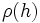# Linearly pushforwardable implies class-preserving for class-separating field

This article gives the statement and possibly, proof, of an implication relation between two automorphism properties. That is, it states that every automorphism satisfying the first automorphism property (i.e., linearly pushforwardable automorphism) must also satisfy the second automorphism property (i.e., class-preserving automorphism)
View all automorphism property implications | View all automorphism property non-implications
Get more facts about linearly pushforwardable automorphism|Get more facts about class-preserving automorphism

## Statement

In a Class-separating field (?), any Linearly pushforwardable automorphism (?) is class-preserving.

## Definitions used

### Class-separating field

Further information: Class-separating field

A field$k$ is termed class-separating for a group$G$ if, given two elements$g,h \in G$ such that:

For every finite-dimensional linear representation$\rho:G \to GL(V)$,$\rho(g)$ and$\rho(h)$ are conjugate in$GL(V)$

Then,$g$ and$h$ are conjugate in$G$.

### Class-preserving automorphism

Further information: class-preserving automorphism

An automorphism of a group is termed class-preserving if it sends every element of the group to an element in its conjugacy class.

### Linearly pushforwardable automorphism

Further information: Linearly pushforwardable automorphism

An automorphism$\sigma$ of a group$G$ is termed linearly pushforwardable over a field$k$ if, for any finite-dimensional linear representation$\rho:G \to GL(V)$, there exists an element$a \in GL(V)$ such that for every$g \in G$, we have:$\rho(\sigma(g)) = a\rho(g)a^{-1}$

## Proof

Given: A group$G$, a class-separating field$k$ for$G$. A linearly extensible automorphism$\sigma$ for$G$.

To prove: For any$g \in G$,$g$ and$\sigma(g)$ are conjugate.

Proof: Let$\rho:G \to GL(V)$ be any finite-dimensional linear representation of$G$ over$k$. Then, since$\sigma$ is linearly pushforwardable, the elements$\rho(g)$ and$\rho(\sigma(g))$ are conjugate inside$GL(V)$.

Since this is true for every finite-dimensional linear representation$\rho$, the definition of class-separating field forces us to conclude that$g$ and$\sigma(g)$ are conjugate.